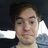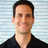Posted over 5 years ago

# Calculating Return on Investment in Real EstateHow do you know if you are getting a good return on your real estate investment? Calculating the ROI on your investment property is critical to knowing how your investment is performing, or when comparing one investment to another.

In order to successfully decide whether a property is worth buying, an investor must run the numbers to calculate two types of returns: Cash-on-cash return on investment, and total return on investment.

Cash on Cash Return on Investment

The cash on cash return on investment is the before-tax cash flow (BTCF) divided by your initial cash investment. The formula looks like this:

Cash on Cash Return on Investment = BTCF / Initial Cash Investment

Your before-tax cash flow is calculated by subtracting your annual mortgage payment from your net operating income (NOI). The net operating income is simply the total income from the property minus the total expenses.

Let’s take a look at an example using a \$150,000 income property purchased with a 20% down payment of \$30,000. Let’s assume your mortgage of \$120,000 is fixed for 30 years at a 7 percent interest rate.

Let’s assume your BTCF is \$3,000 per year (\$250 per month):

Cash on Cash ROI = \$3,000 / \$30,000 = 10.0%

Through the “magic” of leverage using financing to purchase your property, you have created a cash on cash ROI of 10%. This would be quite attractive to most investors in today’s market.

The cash on cash ROI is a good measure of a property’s first year financial performance. However, it does not include the additional benefits achieved through real estate such as the amortization of the mortgage and any future appreciation. The total return on investment addresses that.

Total Return on Investment

The total return on investment (TROI) provides a better and more complete measure of a property’s financial performance. That is because it factors in amortization and appreciation gained over time.

Total ROI = (BTCF + Net Sales Proceeds – Initial Cash Investment) / Initial Cash Investment

In order to calculate the total return on investment, one must project the BTCF for each year of expected ownership as well as the net sales proceeds from the sale of the property.

Let’s take our example above and assume that we plan to sell it in five years with an average annual appreciation rate of 4% per year. After five years our \$150,000 property would be worth \$182,498, and our mortgage balance would be \$111,665. Let’s also assume that our selling expenses total 5% of the sales price, or \$9,125.

Using the figures above, our net sales proceeds from the sale of the property in year five would be \$61,708 (\$182,498 – \$111,665 – \$9,125). Additionally, our before tax cash flow after five years would total \$15,000 assuming no annual increase in rents or cash flow. Now our formula looks like this:

Total Return on Investment = (\$15,000 + \$61,708 – \$30,000) / \$30,000 = 156%

Note that some investors calculate their TROI using their after-tax cash flow (ATCF) instead of the BTCF. This can provide a deeper “bottom line” measure of the return on investment; however, it does not provide a good measure to compare one investment to another since tax liabilities will vary between individual investors. Calculating the TROI using ATCF is best suited for investor specific use.

By projecting a property’s future cash flows and appreciation, you can calculate the potential gains on your initial cash invested (down payment). Assuming the property is not declining in value, the TROI should increase in each successive year.

However, total return on investment can be a little shortsighted when used in isolation. This is because total return on investment does not measure the property’s financial performance as it relates to its equity. For this we must calculate the property’s return on equity (ROE). Similar to the TROI, the return on equity calculation replaces the initial cash invested with the properties equity in a given year. We will take a closer look at ROE in a separate article.

- - - - - - - - - - - - - - - - - - - - - - - - - - - - - - - - - - - - - - - - - - - - - - - - - - - - - - - - - - - - - - - -

1.how do you factor in cap ex risks? isn't it a bit unreasonable to assume no major expenses within 5 years of ownership?

2.Hi @Robert Willix,

Good to hear from you.

There are various articles on our blog at www.NoradaRealEstate.com on these topics, but here are some quick points and rules of thumb:

• Your leveraged cash-on-cash return should be above 10%.
• Total ROI (TROI) should be in the 30% range.
• TROI can be as high as the forty percent range on some properties.

Hope that helps.  Feel free to contact our team for property specific questions.

Continued success,

Marco.

3.Excellent article! I am curious, since I am quite new to this endeavour, if I purchase a rental property, what is the desired ROI? Is there a specific rule of thumb being used as a threshold ROI? I am curious to know your thoughts on the matter. Thanks!